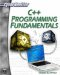# Summary

This chapter has given you an introduction to an important concept in computer science, data structures and algorithms. You have seen how to write a linked list and a bubble sort. The linked list is a very commonly encountered data structure, and the bubble sort is, perhaps, the most commonly encountered sorting algorithm. A careful study of this chapter gives you an introduction to this field of study. Because sorting algorithms are so commonly encountered, it would be of benefit to you to familiarize yourself with the sorting algorithms demonstrated.

This chapter also introduced you to the concept of recursion. This is a simple, but powerful programming concept that is used in a number of situations, including sorting algorithms. You where also shown the Fibonacci sequence, which is a widely studied mathematical curiosity, used frequently in computer science to implement recursive functions.C++ Programming Fundamentals (Cyberrookies)
ISBN: 1584502371
EAN: 2147483647
Year: 2005
Pages: 197
Authors: Chuck Easttom